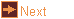# off_trans - transformations (rotations etc) of OFF files

 Usage    |    Examples    |    Notes

## Usage

```

Usage: off_trans [options] [input_file]

Read a file in OFF format and apply transformations to it. If
input_file is not given the program reads from standard input.

Options
-h,--help this help message (run 'off_util -H help' for general help)
--version version information
-T <tran> translate, three numbers separated by commas which are
used as the x, y and z displacements
-R <rot>  rotate about an axis, three, four or six numbers separated by
commas. If three numbers these are angles (degrees) to rotate
about the x, y and z axes. If four numbers, the first three
are a direction vector for the axis, the last number is the
angle (degrees) to rotate. If six numbers, these are two
vectors (from,to) and rotate to carry the first to the second.
If twelve numbers these are four vectors (from1,from2,to1,to2)
and rotate to carry the first onto the third then rotate around
the third to carry the second onto the fourth
-M <norm> reflect in a plane, three numbers separated by commas which
give a vector normal to the plane of reflection.
-S <scal> scale, one, three or four numbers separated by commas. If one
number then scale by this factor in all directions. If three
numbers these are the factors to scale along the x, y and
z axes. If four numbers, the first three are a direction
vector for the scaling, the last number is the factor to scale
-I        inversion
-A <crds> transformation that will align two sets of three points
(18 numbers coordinates of from1,from2,from3,to1,to2,to3)
-a <angs> transformation that makes particular angles between the
mapped axes, angles in degrees in form yz_ang,zx_ang,xy_ang
(corresponding to the angles opposite the x-, y- and z-axis)
-X <mtrx> transformation matrix of 9 or 12 values, given left-to-right
top-to-bottom, used to premultipy each coordinate
-C        translation that carries the centroid to the origin
-y        align geometry with the standard alignment for a symmetry type,
up to three comma separated parts: symmetry subgroup (Schoenflies
notation) or 'full', conjugation type (integer), realignment
(colon separated list of an integer then decimal numbers)
-Y        align standard alignment of a symmetry type with its position
as a subgroup of another symmetry type, up to four comma
separated parts: main symmetry (Schoenflies notation) or
file name, subgroup (Schoenflies notation), conjugation type
(integer), realignment (colon separated list of an integer
then decimal numbers)
-s <type> relative scaling, scale so a measure has a value of 1.
VAa need an oriented polyhedron, V needs a closed polyhedron.
A - area                       a - average face area
E - perimeter (sum of edges)   e - average edge length
V - volume                     r - radius, from centroid
to furthest vertex
-i        replace the current combined transformation by its inverse
-o <file> write output to file (default: write to standard output)

```

## Examples

std_cube is a cube with vertices (±1, ,±1, ±1). To rotate 45 degrees it about the edge running from (1,1,1) to (1,1,-1) the cube must be translated so that line passes through the origin, roatated 45 degrees about the direction of the line, then translated back.

```off_trans -T -1,-1,0 -o cube_a.off std_cube
off_trans -R 0,0,1,45 -o cube_b.off cube_a.off
off_trans -T 1,1,0 -o final_cube.off cube_b.off
antiview final_cube.off
```

This can be combined as
```off_trans -T 1,1,0 -R 0,0,1,45 -T 1,1,0 std_cube | antiview
```

## Notes